WLCheng

•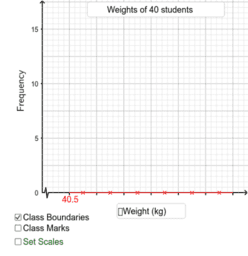Drawing Histograms (Ex.6A, 5)

Activity

WLCheng

•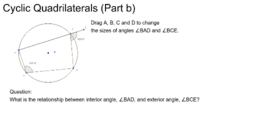Activity

WLCheng

•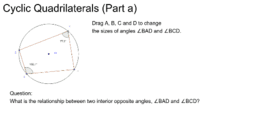Activity

WLCheng

•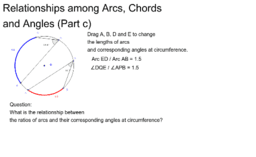Relationships among Ars, Chords and Angles (Part c)

Activity

WLCheng

•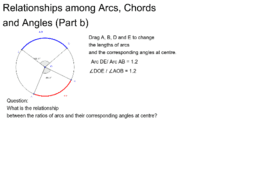Relationships among Ars, Chords and Angles (Part b)

Activity

WLCheng

•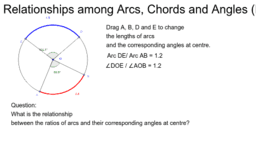Relationships among Ars, Chords and Angles (Part b)

Activity

WLCheng

•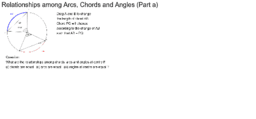Relationships among Arcs, Chords and Angles(Part a)

Activity

WLCheng

•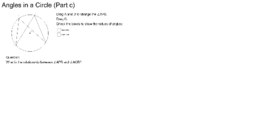Angles in a circle (Part c)

Activity

WLCheng

•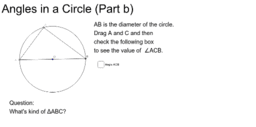Angles in a circle (Part b)

Activity

WLCheng

•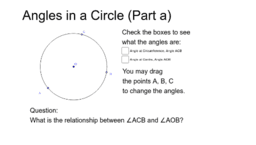Angles in a Circle (Part a)

Activity

WLCheng

•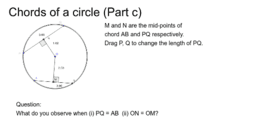Chords of a circle (Part c)

Activity

WLCheng

•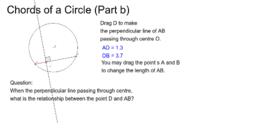Chords of a Circle (Part b)

Activity

WLCheng

•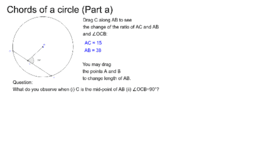Angles in a circle

Activity

WLCheng

•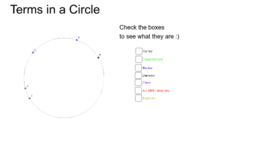Terms in a circle

Activity

WLCheng

•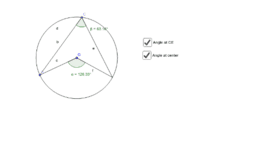Activity

WLCheng